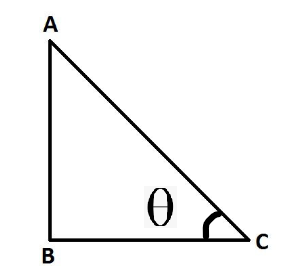GeeksforGeeks App
Open AppBrowser
Continue

# Prove that 1/(sin θ + cos θ) + 1/(sin θ – cos θ) = 2 sin θ/(1 – 2 cos2 θ)

Trigonometry is a branch of mathematics that generally deals with triangles and angles associated with it. Trigonometry helps in understanding the properties of triangles and the real-life application of the triangle properties. Trigonometry consists of two words namely trigon and geometry, which itself tells the meaning in simple form i.e. geometry of triangles (where trigon refers to triangles).

Trigonometry helps in understanding how to find missing angles provided the length of the sides of triangles are given, or it can also be used to find the value of certain angles like 0,30,45,60,90, etc.

### Trigonometric Ratios

There are six functions or trigonometric ratios of an angle that are present in trigonometry. Their names and abbreviations are sine (sin), cosine (cos), tangent (tan), cotangent (cot), secant (sec), and cosecant (csc). One important thing to note here is that trigonometric formulas work only on the right-angle triangle. Let’s see the below image:In this right-angle triangle, side AC is known as hypotenuse. Side BC is known as the base of the triangle. Side AB is known as the height of the triangle. From the above triangle, we can list out formulas as above,

• sin∅ = AB/AC
• cos∅ = BC/AC
• tan∅ = AB/BC
• cot∅ = BC/AB
• cosec∅ = AC/AB
• sec∅ = AC/BC

Basic formulas of trigonometric ratios

• Tan ∅ = sin ∅/cos ∅
• sin ∅ = 1/cosec ∅
• cos ∅ = 1/sec ∅
• Tan ∅ = 1/cot ∅
• sin(90° – x) = cos x
• cos(90° – x) = sin x
• tan(90° – x) = cot x
• cot(90° – x) = tan x
• cos2x+sin2x=1
• 1 + cot2θ = cosec2θ
• 1 + tan2θ = sec2θ

### Prove that 1/(sin θ + cos θ) + 1/(sin θ – cos θ) = 2 sin θ/(1 – 2 cos2 θ)

Proof:

Taking the LHS of the equation,

1/(sin θ + cos θ) + 1/(sin θ – cos θ)

= (sinθ – cos θ+sin θ + cos θ)/(sin2 θ – cos2 θ) [taking lcm of the equations]

= 2sinθ/(sin2 θ – cos2 θ)

in the RHS, the denominator is in cos terms. so, substituting sin2θ = 1 – cos2θ from the formulas,

= 2sinθ/(1- 2cos2 θ)

= RHS

### Similar Questions

Question 1: Prove that (1 – cos2θ)cosec2θ = 1

Solution:

Taking the LHS of the equation,

(1-cos2 θ)cosec2θ

= (sin2θ)cosec2θ   [as cos2x + sin2x = 1]

= cosec2θ /cosec2θ   [as sin2θ = 1/cosec2θ]

= 1

= RHS

Question 2: Prove that sec2θ + tan2θ = 2secθ – 1

Solution:

Taking the LHS of the equation,

sec2θ + tan2θ

= 1/cos2θ  + sin2θ/cos2θ  [ as cos ∅ = 1/sec ∅ and Tan ∅ = sin ∅/cos ∅]

= (1+sin2θ)/cos2θ   [taking lcm]

= (1+1-cos2θ )/cos2θ   [as cos2x+sin2x = 1]

= (2-cos2θ )/cos2θ

= 2/cos2θ – cos2θ/cos2θ  [splitting the denominator]

= 2sec2θ -1

= RHS

Question 3: Prove that cotθ + cosecθ = (cosθ + 1)/sinθ

Solution:

Taking the LHS of the equation,

cotθ + cosecθ

= cosθ/sinθ +1/sinθ  [as sin ∅ = 1/cosec ∅ and cot∅ =cos∅ /sin∅ ]

= (cosθ+1)/sinθ   [taking lcm]

= RHS

Question 4: Prove that tanθ.sinθ + secθ.cos2θ = secθ

Solution:

Taking the LHS of the equation,

tanθ.sinθ + secθ.cos2θ

= sinθ.sinθ/cosθ + cos2θ/cosθ  [as cos ∅ = 1/sec ∅ and Tan ∅ = sin ∅/cos ∅]

= (sin2θ + cos2θ)/cosθ  [as cos2x+sin2x = 1]

= 1/cosθ

= secθ

= RHS

My Personal Notes arrow_drop_up
Related Tutorials